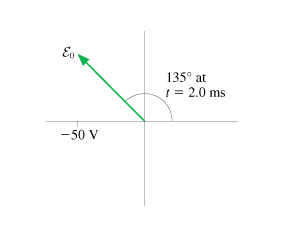# Problem: The emf phasor in the figure is shown at t = 2.0 ms.What is the angular frequency?Assume this is the first rotation. What is the peak value of the emf?

###### FREE Expert Solution
80% (354 ratings)###### Problem Details

The emf phasor in the figure is shown at t = 2.0 ms.What is the angular frequency?

Assume this is the first rotation. What is the peak value of the emf?